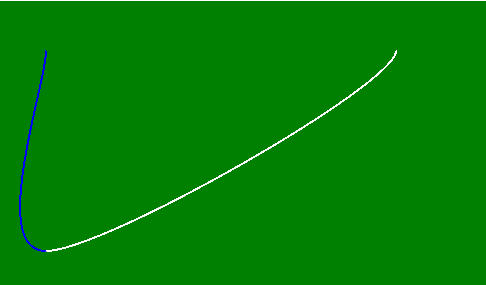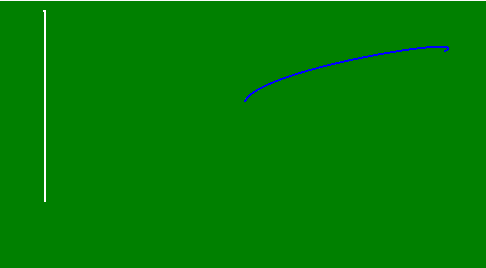# p5.js | curve() function

The curve() function is used to draws a curved line between two points given in the middle four parameters on the screen. The first two and last two parameters are used as a control point.

Syntax:

`curve( x1, y1, x2, y2, x3, y3, x4, y4 )`

or

`curve( x1, y1, z1, x2, y2, z2, x3, y3, z3, x4, y4, z4 )`

Parameters:

Value Description
x1 It is used to hold the x-coordinate of beginning control point.
y1 It is used to hold the y-coordinate of beginning control point.
z1 It is used to hold the z-coordinate of beginning control point.
x2 It is used to hold the x-coordinate of first point.
y2 It is used to hold the y-coordinate of first point.
z2 It is used to hold the z-coordinate of first point.
x3 It is used to hold the x-coordinate for second point.
y3 It is used to hold the y-coordinate for second point.
z3 It is used to hold the z-coordinate for second point.
x4 It is used to hold the x-coordinate of ending control point.
y4 It is used to hold the y-coordinate of ending control point.
z4 It is used to hold the z-coordinate of ending control point.

Below examples illustrate the curve() function in CSS:

Example 1:

 `function setup() {  ` `   `  `    ``// Create canvas of given size ` `    ``createCanvas(500, 300);  ` `   `  `    ``// Set the background of canvas ` `    ``background('green');  ` `}  ` ` `  `function draw() {  ` `     `  `    ``// Use noFill() function to not fill the color ` `    ``noFill(); ` `   `  `    ``// Set the stroke color ` `    ``stroke('white'); ` `   `  `    ``// Use curve() function to create curve ` `    ``curve(50, 50, 400, 50, 50, 250, 50, 50); ` ` `  `    ``// Set the stroke color ` `    ``stroke('blue'); ` `   `  `    ``// Use curve() function to create curve ` `    ``curve(400, 50, 50, 250, 50, 50, 50, 50);  ` `}  `

Output:Example 2:

 `function setup() {  ` `   `  `    ``// Create canvas of given size ` `    ``createCanvas(500, 300);  ` `   `  `    ``// Set the background of canvas ` `    ``background('green');  ` `}  ` ` `  `function draw() {  ` `     `  `    ``// Use noFill() function to not fill the color ` `    ``noFill(); ` `   `  `    ``// Set the stroke color ` `    ``stroke('white'); ` `   `  `    ``// Use curve() function to create curve ` `    ``curve(50, 50, 50, 200, 50, 10, 50, 250, 150, 50, 50, 50); ` ` `  `    ``// Set the stroke color ` `    ``stroke('blue'); ` `   `  `    ``// Use curve() function to create curve ` `    ``curve(50, 200, 450, 50, 250, 100, 350, 250, 250, 450, 450, 400);  ` `}  `

Output:My Personal Notes arrow_drop_upCheck out this Author's contributed articles.

If you like GeeksforGeeks and would like to contribute, you can also write an article using contribute.geeksforgeeks.org or mail your article to contribute@geeksforgeeks.org. See your article appearing on the GeeksforGeeks main page and help other Geeks.

Please Improve this article if you find anything incorrect by clicking on the "Improve Article" button below.

Article Tags :

Be the First to upvote.

Please write to us at contribute@geeksforgeeks.org to report any issue with the above content.